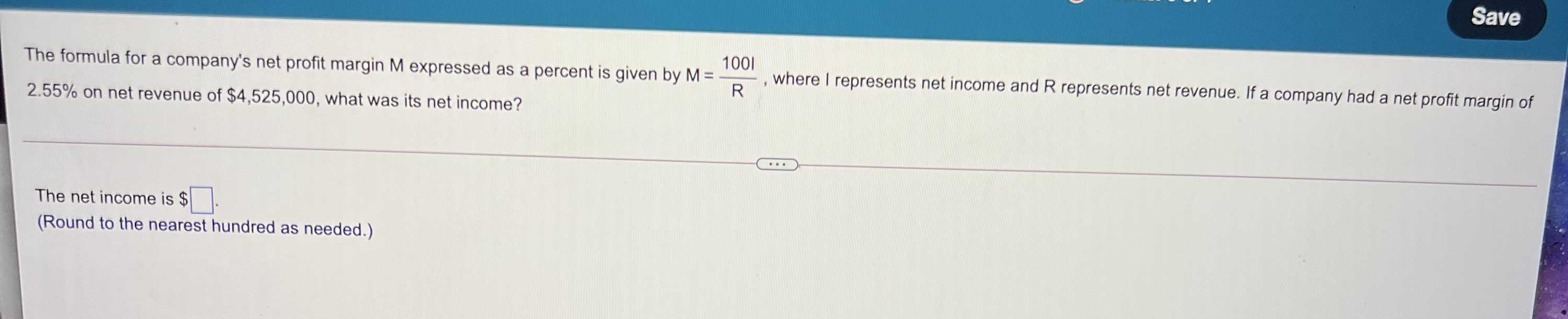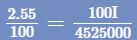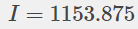### Still have math questions?

Algebra
QuestionThe formula for a company's net profit margin M expressed as a percent is given by $$M = \frac { 1001 } { R }$$ , where I represents net income and $$R$$ represents net revenue. If a company had a net profit margin of $$2.55 \%$$ on net revenue of $$\ 4,525,000$$ , what was its net income?

The net income is $$\ \square$$ . (Round to the nearest hundred as needed.)